# Playing With Numbers Solution of TS & AP Board Class 8 Mathematics

#### Playing With Numbers Solution of  TS & AP Board Class 8 Mathematics

###### Exercise 15.1

Question 1.

Using divisibility rules, find which of the following numbers are divisible by 2,5,10 (say yes or no ) in the given table . What do you observe?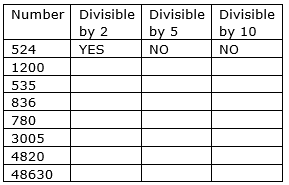From the divisibility rules we know that if the given number ends with 0 then it is divisible by 10 and if the number ends with 0 or 5 it is divisible by 5 and if the unit place of a number is divisible by 2 then the number is divisible by 2.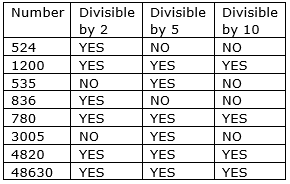We observe that if a number is divisible by 10 then the number is also divisible by 2 and 5.

Question 2.

Using divisibility tests, determine which of following numbers are divisible by 2

(a) 2144 (b) 1258

(c) 4336 (d) 633 (e) 1352

(a) 2144 is divisible by 2. Since, the unit place of the number is 4 which is divisible by 2.

(b) 1258 is divisible by 2. Since, the unit place of the number is 8 which is divisible by 2.

(c) 4336 is divisible by 2. Since, the unit place of the number is 6 which is divisible by 2.

(d) 633 is not divisible by 2 because unit place of the number is 3.

(e) 1352 is divisible by 2. Since, the unit place of the number is 2 which is divisible by 2.

Question 3.

Using divisibility tests, determine which of the following numbers are divisible by 5

(a) 438750 (b) 179015

(c) 125 (d) 639210 (e) 17852

(a) 438750 is divisible by 5. Since, the given number ends with 0.

(b) 179015 is divisible by 5. Since, the given number ends with 5.

(c) 125 is divisible by 5. Since, the given number ends with 5

(d) 639210 is divisible by 5. Since, the given number ends with 0

(e) 17852 is not divisible by 5.

Question 4.

Using divisibility tests, determine which of the following numbers are divisible by 10:

(a) 54450 (b) 10800

(c) 7138965 (d) 7016930 (e) 10101010

(a) 54450 is divisible by 10. Since, the given number ends with 0.

(b) 10800 is divisible by 10. Since, the given number ends with 0.

(c) 7138965 is not divisible by 10.

(d) 7016930 is divisible by 10. Since, the given number ends with 0.

(e) 10101010 is divisible by 10. Since, the given number ends with 0.

Question 5.

Write the number of factors of the following?

18

18

Prime factors of 18 are = 2 × 3 × 3

= 21 × 32

⇒ Now, number of factors can be written as n + 1 form

⇒ (1 + 1)(2 + 1) = 2 × 3 = 6

Hence, number of factors for 18 is 6

Question 6.

Write the number of factors of the following?

24

24

Prime factors of 24 are = 2 × 2 × 2 × 3

= 23 × 31

⇒ Now, number of factors can be written as n + 1 form

⇒ (3 + 1)(1 + 1) = 4 × 2 = 8

Hence, number of factors for 24 is 8

Question 7.

Write the number of factors of the following?

45

45

Prime factors of 45 are = 5 × 3 × 3

= 51 × 32

⇒ Now, number of factors can be written as n + 1 form

⇒ (1 + 1)(2 + 1) = 2 × 3 = 6

Hence, number of factors for 45 is 6

Question 8.

Write the number of factors of the following?

90

90

Prime factors of 90 are = 3 × 3 × 2 × 5

= 51 × 32 × 21

⇒ Now, number of factors can be written as n + 1 form

⇒ (1 + 1)(2 + 1)(1 + 1) = 2 × 3 × 2 = 12

Hence, number of factors for 90 is 12

Question 9.

Write the number of factors of the following?

105

105

Prime factors of 105 are = 3 × 7 × 5

= 51 × 31 × 71

⇒ Now, number of factors can be written as n + 1 form

⇒ (1 + 1)(1 + 1)(1 + 1)

= 2 × 2 × 2 = 8

Hence, number of factors for 105 is 8

Question 10.

Write any 5 numbers which are divisible by 2, 5 and 10.

Need to find out 5 numbers which are divisible by 2, 5 and 10

We know from the divisibility rules that any number which is divisible by 10 is divisible by 2 and 5.

∴ we should take numbers which ends with 0.

⇒ 10,20,30,40,50,60.. are the numbers which are divisible by 2,5 and 10.

Question 11.

A number 34A is exactly divisible by 2 and leaves a remainder 1, when divided by 5, find A.

Given, a number 34A

Need to find out it must be divisible by 2 and when it divides with 5 it leaves the remainder 1

⇒ For a number to be exactly divisible by 2 the unit place of a number must be divided by 2.

So, by trial and error method number ending with 2,4, 6 are divisible by 2 but it should be divisible by 5 to get a remainder 1.

∴ if A = 6 then the number will be 346 which is divisible by 2 and also by 5 by leaving a remainder 1.

Hence, A = 6 and the number is 346.

###### Exercise 15.2

Question 1.

If 345 A 7 is divisible by 3, supply the missing digit in place of ‘A’.

Given, a number 345A7 divisible by 3

Need to find out A

⇒ Sum of the digits of 345A7 must be divisible by 3

3 + 4 + 5 + A + 7 = 19 + A must be divided by 3

Substitute A value from 0 to 9 by trial error method, we get

A might be 2 or 5 or 8

If A = 2 then 19 + 2 = 21 is divisible by 3.

If A = 5 then 19 + 5 = 24 is divisible by 3.

If A = 8 then 19 + 8 = 27 is divisible by 3.

Hence, A might be 2 or 5 or 8

Question 2.

If 2791 A, is divisible by 9, supply the missing digit in place of ‘A’.

Given, 2791A a number divisible by 9

Need to find out A

⇒ Sum of the digits of a number 2791A must be divisible by 9

⇒ 2 + 7 + 9 + 1 + A = 19 + A must be divisible by 9

Substitute A value from 0 to 9 by trial error method

We get

A = 8 as 27918 is divisible by 9.

19 + 8 = 27 is divisible by 9.

Hence, A is 8.

Question 3.

Write some numbers which are divisible by 2,3,5,9 and 10 also.

Need to write the numbers which are divisible by 2,3,5,9,10

Numbers which are divisible by 10 are divisible by 2 and 5

And numbers which are divisible by 9 are divisible by 3

∴ numbers are 90, 180, 270, 360, 450

Question 4.

2A8 is a number divisible by 2, what might be the value of A?

Given, a number 2A8 divisible by 2

Need to find out the value of A

The value might be 0 to 9. As, the divisibility of the number does not depend on tens place of a number

Any value can be satisfied as unit place number is divisible by 2

Question 5.

50B is a number divisible by 5, what might be the value of B?

Given, a number 50B divisible by 5

Need to find out the value of B

⇒ 50B is divisible by 5 if the unit place is either 0 or 5

Hence, the value of B might be 0 or 5

Question 6.

2P is a number which is divisible by 2 and 3, what is the value of P

Given, a number 2P divisible by 2 and 3

Need to find out the value of P

⇒ 2P is divisible by 2 if unit place is divisible by 2

P can be 2,4,6

But it should be divisible by 3 also

So, P = 4

24 is divisible by both 2 and 3

Hence, P is 4.

Question 7.

54Z leaves remainder 2 when divided by 5, and leaves remainder 1 when divided by 3, what is the value of Z?

Given, 54Z a number

54Z divided by 5 leaves a remainder 2

And 54Z divided by 3 leaves a remainder 1

Need to find out the value of Z

Substitute Z value from trial error method from 0 to 9 satisfying the above conditions, we get

⇒ Z as 7

Since, 54Z = 547 divided by 5 leaves remainder 2

And 547 divided by 3 leaves remainder 1

Hence, Z is 7.

Question 8.

27Q leaves remainder 3 when divided by 5 and leaves remainder 1 when divided by 2, what is the remainder when it is divided by 3 ?

Given, 27Q leaves a remainder 3 when divided by 5 and leaves remainder 1 when divided by 2

Need to find out the remainder when divided by3

Substitute Q value from trial error method from 0 to 9

⇒ Q = 3 when substituted in the given number

⇒ 27Q = 273 is divided by 5 and get the remainder as 3

⇒ 273 is divided by 2 and get the remainder as 1

Satisfies the above equation.

⇒ 273 is divided by 3 and we get the remainder as 0.

Hence, when 27Q = 273 is divided by 3 we get 0 as the remainder.

###### Exercise 15.3

Question 1.

Check whether the given numbers are divisible by ‘6’ or not?

(a) 273432 (b) 100533

(c) 784076 (d) 24684

(a), (d) are divisible by 6

For a number to be divisible by 6 it must be divisible by 2 and 3

(a) 273432 is divisible by 2. Since, unit place of a number is divided by 2

⇒ 273432 is divisible by 3.

Since, sum of digits of a number = 2 + 7 + 3 + 4 + 3 + 2 = 21 is divisible by 3.

(b) 100533 is not divisible by 2.

∴ it is not divisible by 6.

(c) 784076 is not divisible by 2.

∴ it is not divisible by 6.

(d) 24684 is divisible by 2. Since, unit place of a number is divided by 2

⇒ 24684 is divisible by 3.

Since, sum of digits of a number = 2 + 4 + 6 + 8 + 4

= 24 is divisible by 3.

Question 2.

Check whether the given numbers are divisible by ‘4’ or not?

(a) 3024(b) 1000

(c) 412 (d) 56240

All are divisible by 4

From the divisibility rule we have (10c + d) is divisible by 4

(a) 3024 is divisible by 4.

Since, (10(2) + 4) = 24 is divisible by 4.

(b) 1000 is divisible by 4.

Since, (10(0) + 0) = 0 is divisible by 4.

(c) 412 is divisible by 4.

Since, (10(1) + 2) = 12 is divisible by 4.

(d) 56240 is divisible by 4.

Since, (10(4) + 0) = 40 is divisible by 4.

Question 3.

Check whether the given numbers are divisible by ‘8’ or not?

(a) 4808(b) 1324

(c) 1000 (d) 76728

(a), (c), (d) are divisible by 8

From the divisibility rule we have (100(b) + 10c + d) is divisible by 8

(a) 4808 is divisible by 8.

Since, number formed by last three digits is divisible by 8

(b) 1324 is divisible by 8.

Since, number formed by last three digits is not divisible by 8

(c) 1000 is divisible by 8.

0 is divisible by 8

(d) 76728 is divisible by 8.

Since, number formed by last three digits is divisible by 8

Question 4.

Check whether the given numbers are divisible by ‘7’ or not?

(a) 427 (b) 3514

(c) 861 (d) 4676

All the given numbers are divisible by 7

From divisibility rules we have (2a + 3b + c)

(a) 427 is divisible by 7

Since, (2(4) + 3(2) + 7) = 8 + 6 + 7 = 21 is divisible by 7

(b) 3514 is divisible by 7

Since, (6(3) + 2(5) + 3(1) + 4) = 18 + 10 + 3 + 4 = 35 is divisible by 7

(c) 861 is divisible by 7

Since, (2(8) + 3(6) + 1) = 16 + 18 + 1 = 35 is divisible by 7

(d) 4676 is divisible by 7

Since, (6(4) + 2(6) + 3(7) + 6) = 24 + 12 + 21 + 6 = 63 is divisible by 7

Question 5.

Check whether the given numbers are divisible by ’11’ or not?

(a) 786764 (b) 536393

(c) 110011 (d) 1210121

(e) 758043 (f) 8338472

(g) 54678 (i) 13431

(j) 423423 (k) 168861

(a), (b), (c), (d), (e), (i), (j) and (k) are divisible by 11

(a) 786764 is divided by 11 if difference between sum of odd numbers and sum of even numbers is 0 or 11.

⇒ (7 + 6 + 6)-(8 + 7 + 4) = 19-19 = 0

(b) 536393 is divided by 11 if difference between sum of odd numbers and sum of even numbers is 0 or 11.

⇒ (5 + 6 + 9)-(3 + 3 + 3) = (20)-9 = 11

(c) 110011 is divided by 11 if difference between sum of odd numbers and sum of even numbers is 0 or 11.

⇒ (1 + 0 + 1)-(1 + 0 + 1) = 0

(d) 1210121 is divided by 11 if difference between sum of odd numbers and sum of even numbers is 0 or 11.

⇒ (1 + 1 + 1 + 1)-(2 + 0 + 2) = 0

(e) 758043 is divided by 11 if difference between sum of odd numbers and sum of even numbers is 0 or 11.

⇒ (7 + 8 + 4)-(5 + 0 + 3) = 19-8 = 11

(i) 13431 is divided by 11 if difference between sum of odd numbers and sum of even numbers is 0 or 11.

⇒ (1 + 4 + 1)-(3 + 3) = 0

(j) 423423 is divided by 11 if difference between sum of odd numbers and sum of even numbers is 0 or 11.

⇒ (4 + 3 + 2)-(2 + 4 + 3) = 0

(k) 168861 is divided by 11 if difference between sum of odd numbers and sum of even numbers is 0 or 11.

⇒ (1 + 8 + 6)-(6 + 8 + 1) = 0

(g) 54678 is not divisible by 11 since the difference between sum of odd numbers and sum of even numbers is not 0 or 11.

⇒ (5 + 6 + 8)-(4 + 7) = 19-11 = 8

Question 6.

If a number is divisible by ‘8’, then it also divisible by ‘4’ also. Explain?

Given, a number is divisible by ‘8’ it is also divisible by ‘4’

All multiples of 8 are also the multiples of 4 and if a number is divided by 8 then it is also divisible by 4 because 8 is divisible by 4.

Example:

Let us consider a number 32

Which is a multiple of 8,4 and can be divided by 8 and also by

4.

Question 7.

A 3-digit number 4A3 is added to another 3-digit number 984 to give four digit number 13B7, which is divisible by 11. Find (A + B).

Given, a 3-digit number 4A3

And a 3-digit number 984

Both the numbers are added to get a four-digit number 13B7

And 13B7 is divisible by 11.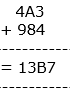By observation we get A + 8 = B

⇒ B-A = 8

Since, 13B7 is divisible by 11 to know its divisibility we have to subtract sum of odd placed numbers and sum of even placed numbers

We get,

(7 + 3)-(B + 1) = 9-B = 0

⇒B = 9

Solving, B in B-A = 8 we get

⇒ 9-A = 8

⇒ A = 9-8

⇒ A = 1

Hence, A = 1, B = 9 and (A + B) = 10

###### Exercise 15.4

Question 1.

Check whether 25110 is divisible by 45.

Given, a number 25110

Need to find out the number is divisible by 45

⇒ The prime factorization of 45 = 5 × 9

So, it is sufficient to find the number divisible with 5 and 9

⇒ 25110 is divisible by 5 as ‘0’ is at its units place

⇒ the sum of the digits of a number = 2 + 5 + 1 + 1 + 0 = 9 which is divisible by 9

Hence, the given number 25110 is divisible by 45.

Question 2.

Check whether 61479 is divisible by 81.

Given, number is 61479.

Need to find out the number is divisible by 81

⇒ Prime factors of 81 = 9 × 9

∴ it is enough to find the number is divisible by 9

⇒ To test the number is divisible by 9 it can be found by summing of the digits

⇒ 6 + 1 + 4 + 7 + 9 = 27

∴ number 27 is divisible by 9

Hence, 61479 is divisible by 81.

Question 3.

Check whether 864 is divisible by 36? Verify whether 864 is divisible by all the factors of 36?

Given, a number 864

Need to find out the number is divisible by 36

⇒ Prime factors of 36 = 2,2,3,3

So, it is sufficient to find the given number is divisible by 2 and 3.

⇒ Units place in 864 is even number and is divisible by 2 which is also even number.

∴ 864 is divisible by 2

⇒ sum of digits of 864 is 8 + 6 + 4 = 18

⇒ 18 is divisible by 3

∴ 864 is divisible by 3

Hence, 864 is divisible by 2 and 3.

Question 4.

Check whether 756 is divisible by 42? Verify whether 756 is divisible by all the factors of 42?

Given, a number is 756

Need to find out the given number is divisible by 42 and its factors.

⇒ Prime factors of 42 = 7,6

⇒ to test the number is divisible by 6 we need to sum the digits of 756

⇒ 7 + 5 + 6 = 18 is divisible by 6

⇒ to test the number is divisible by 7

⇒ Now, (2a + 3b + c) is divisible by 7

⇒ 2(7) + 3(5) + 6 = 14 + 15 + 6 = 35 is divisible by 7

Hence, 756 is divisible by 7

Question 5.

Check whether 2156 is divisible by 11 and 7? Verify whether 2156 is divisible by product of 11 and 7?

Given, a number is 2156

Need to find out the given number is divisible by 11 and 7

Also, need to check number divisible by product of 11 and 7.

⇒For, the number 2156 the difference of the digits of odd places and even places must be divisible by 11 or it must be 0.

⇒ (2 + 5)-(1 + 6) = 7-7 = 0

∴ the number is divisible by 11

⇒ Now, we know (6a + 2b + 3c + d) by divisibility rule

⇒ 6(2) + 2(1) + 3(5) + 6

= 12 + 2 + 15 + 6 = 35

∴ 35 is divisible by 7

Product of 11, 7 = 77 which is divisible by 7

Hence, 2156 is divisible by 11 and 7 and divisible by product of 11 and 7.

Question 6.

Check whether 1435 is divisible by 5 and 7? Verify if 1435 is divisible by the product of 5 and 7?

Given, “1435” a number.

Need to find out it is divisible by 5 and 7 and also by product 5 × 7

⇒ 1435 is divisible by 5. Since, units digit of the number is 5.

⇒ Now, we know (6a + 2b + 3c + d) by divisibility rule

⇒ 6(1) + 2(4) + 3(3) + 5 = 6 + 8 + 9 + 5

= 28 is divisible by 7

∴ 1435 is divisible by 7

The product of 5,7 is 35

∴ 1435 is divisible by 35 because it is divisible by 5 and divisible by 7.

Hence, 1435 is divisible by 5 and 7 and divisible by the product of 5 and 7.

Question 7.

Check whether 456 and 618 are divisible by 6? Also check whether 6 divides the sum of 456 and 618?

Given, “456” and “618” two numbers.

Need to find out it is divisible by 6 and also sum of the two numbers is divisible by 6.

⇒ prime factors of 6 are 2,3

⇒ 456 is divisible by 2 since, units place of a number’456’ is 6 which is divisible by 2.

⇒ Sum of the digits of 456 must be divisible by 3

⇒ 4 + 5 + 6 = 15 is divisible by 3.

∴ 456 is divisible by 6.

⇒ 618 is divisible by 2. Since, units place of a number ‘618’ is 8 which is divisible by 2.

⇒ Sum of the digits of 618 must be divisible by 3.

⇒ 6 + 1 + 8 = 15 is divisible by 3.

∴ 618 is divisible by 6.

Sum of 456, 618 = 456 + 618

= 1074 is divisible by 6 needs to be calculated

⇒ 1074 is divisible by 2 since, unit place of a number ‘1074’ is 4 which is divisible by 2.

⇒ Sum of the digits of 1074 must be divisible by 3.

⇒ 1 + 0 + 7 + 4 = 12 is divisible by 3.

∴ 1074 is divisible by 6

Hence, 456,618 and their sum is divisible by 6.

Question 8.

Check whether 876 and 345 are divisible by 3? Also check whether 3 divides the difference of 876 and 345?

Given, “876” and “345” two numbers.

Need to find out they are divisible by 3 and also their difference divides 3 should be found out.

⇒ sum of the digits of 876 is 8 + 7 + 6 = 21 is divisible by 3.

⇒ sum of the digits of 345 is 3 + 4 + 5 = 12 is divisible by 3.

⇒ difference of 876 and 345 = 876 -345 = 531

⇒ Sum of the digits of 531 = 5 + 3 + 1 = 9 is divisible by 3.

Hence, 876 and 345 are divisible by 3 and the difference between them is also divisible by 3.

Question 9.

Check whether 22 + 23 + 24 is divisible by 2 or 4 or by both 2 and 4?

Given, 22 + 23 + 24

Need to calculate it is divisible by 2 or 4 or both

⇒ 22 + 23 + 24 can be written as

⇒ 4 + 8 + 32 = 44 is divisible by 2 or 4 or by both since, unit place of a number is divisible by 2 or 4 or both.

Hence, the number is divisible by both 2 and 4

Question 10.

Check whether 322 is divisible by 4 or 8 or by both 4 and 8?

Given, 322

Need to find out it is divisible by 4 or 8 or both.

⇒ 322 can be written as 1024

⇒ Now, by divisibility rule we have (10c + d) must be divisible by 4

⇒ 1024 is divisible by 4. Since, 10(2) + 4 = 24 is divisible by 4.

⇒ Now, by divisibility rule we have (100b + 10c + d) must be divisible by 8.

⇒ 1024 is divisible by8. Since, 100(0) + 10(2) + 4 = 24 is divisible by 8.

Hence, 322 is divisible by both 4 and 8.

Question 11.

If A679B is a 5-dit number is divisible by 72 find ‘A’ and ‘B’?

Given, A679B a number.

Need to find out it is divisible by 72.

⇒ Prime factors of 72 = 9 and 8

So, it is enough to check the given 5-digit number is divisible by 9 and 8.

⇒ To check the number is divisible by 8 last three digits must be divisible by 8.

⇒ 79B is divisible by 8 should be checked.

⇒ Now, from divisibility rule 100b + 10c + d

We get

⇒ 100(7) + 10(9) + B = 790 + B

Substitute a number in B which satisfy the equation using trail error method

⇒ B must be 2 to be divisible by 8

⇒ 792 is divisible by 8

∴ A679B = A6792 is divisible by 8

⇒ A6792 is divisible by 9 only if sum of the given digits is divisible by 9

⇒ A + 6 + 7 + 9 + 2 = A + 24

Put a value in A which satisfy the equation

A = 3

A + 24 = 27 is divisible by 9.

∴ A679B is divisible by 72 and the values of A = 3 and B = 2

Hence, 36792 is divisible by 72

###### Exercise 15.5

Question 1.

Find the missing digits in the following additions.

(a)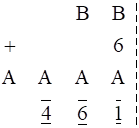(b)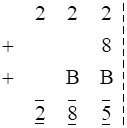(c)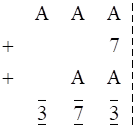(d)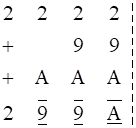(e)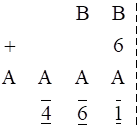(a) Observing the equation we get A = 9

(b) Observing the equation we get B = 5

(c) Observing the equation we get A = 3

(d) Observing the equation we get A = 6 and sum is 2996

(e) Observing the equation we get A = 4, B = 1

By trial and error method we solve the equations.

Question 2.

Find the value of A in the following

(a) 7A – 16 = A9 (b) 107 – A9 = 1A

(c) A36 – 1A4 = 742

(a) 7A – 16 = A9

⇒ Solve this equation by using the numbers from 0,1,2…… in A

Finally, 5 solves the equation as

75 – 16 = 59

Hence, A is 5

(b) 107-A9 = 1A

⇒ Solve this equation by using the numbers from 0,1,2…… in A

Finally, 8 solves the equation as

107-89 = 77

Hence, A is 77

(c) A36 – 1A4 = 742

⇒ Solve this equation by using the numbers from 0,1,2…… in A

Finally,9 solves the equation

936-194 = 742

Hence, A is 9

Question 3.

Find the numerical value of the letters given below-

(a)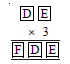(b)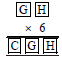(a) Given, product of DE and 3 = FDE

Since DE is multiple of 3 we need to find the number such that numbers D, E exists in the product value. By trial and error method we get

D = 5 and E = 0

And the product of 50 × 3 = 150

∴ F = 1 and D, E numbers exists in the value 150

Hence, D = 5, E = 0 and F = 1

(b) Given, Product of GH and 6 = CGH

Since GH is multiple of 6 we need to find the number such that numbers G, H exists in the product value. By trial and error method we get

G = 2 and H = 0

And the product of 20 × 6 = 120

∴ C = 1 and G, H numbers exists in the value CGH

Hence, C = 1, G = 2 and H = 0

Question 4.

Replace the letters with appropriate digits

(a) 73K ÷ 8 = 9L (b) 1MN ÷ 3 = MN

(a) Given, 73K ÷ 8 = 9L

Need to find out K, L

Here, remainder is not in a decimal form.

So, we need to substitute K with the values from 1,2,3…till we reach a number such that it is exactly divisible by 8.

∴ K = 6

Substituting the value, we get 736 ÷ 8 = 92

∴ L = 2

Hence, K = 6 and L = 6

(b) Given, 1MN ÷ 3 = MN

Need to find the values of M and N

Here, remainder is not in a decimal form and M, N exists in the resultant value after it is divided by 3

So, we need to substitute M, N with the values from 0,1,2,3…till we reach a number such that it is exactly divisible by 3.

∴ M = 5 and N = 0

150 ÷ 3 = 50

Hence, M = 5 and N = 0

Question 5.

If ABB × 999 = ABC123 (where A, B, C are digits) find the values of A, B, C.

Given, product of ABB and 999 = ABC123

Need to find out the values of A, B, C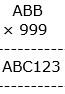We know that 7 × 9 = 63

So, ABB must be a number ending with 7

⇒ A77 × 999 = ABC123

Let us consider it A77 be 877 by trial and error method

∴ 877 × 999 = 876123

⇒ ABC123 = 876123

∴ A = 8, B = 7 and C = 6

###### Exercise 15.6

Question 1.

Find the sum of integers which are divisible by 5 from 1 to 100.

Given, Sum of integers from 1 to 100

Need to find out the numbers which are divisible by 5.

The numbers which are divisible by 5 are 5,10,15…..100

⇒ Now, Sum of integers form 1 to N =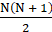Sum of integers from 1 to 100 divisible by 5

= (5 + 10 + ….. + 100)

= 5(1 + 2 + …. + 20)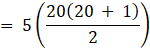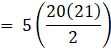= (5 × 10 × 21)

= 1050

Hence, the sum of integers which are divisible by 5 from 1 to 100 is 1050.

Question 2.

Find the sum of integers which are divisible by 2 from 11 to 50.

Given, Sum of integers from 11 to 50

we need to find out the numbers which are divisible by 2

⇒ Now, Sum of integers form 1 to N =⇒ Sum of integers divisible by 2 from 11 to 50 = Sum of integers divisible by 2 from 1 to 50 – Sum of integers divisible by 2 from 1 to 49

= (2 + 4 + 6………. + 50) – (2 + 4 + 6…. + 10)

= 2(1 + 2 + 3…… + 25)-2(1 + 2 + .. + 5)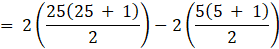= (25 × 26)-(5 × 6)

= 650 – 30

= 620

Hence, the sum of integers which are divisible by 2 from 11 to 50 is 620

Question 3.

Find the sum of integers which are divisible by 2 and 3 from 1 to 50.

Given, Sum of integers from 1 to 50

Need to find out the numbers which are divisible by 2 and 3.

Here, since the number should be divisible by 2 and also 3 it is enough to find the sum of integers which are divisible by 6.

∴ the sum of numbers which are divisible by 6 from 1 to 50 = 6,12,18…48

⇒ Now, Sum of integers form 1 to N == (6 + 12 + 18 + ….. + 48)

= 6(1 + 2 + 3 + …. + 8)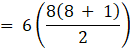= 3(8 × 9)

= 216

Hence, the sum of integers from 1 to 50 which are divisible by 2 and 3 is 216.

Question 4.

(n3 − n) is divisible by 3. Explain the reason.

Given, (n3 – n) is divisible by 3

Here, (n3 – n) can be written as n(n2 -1)

⇒ n(n2 -12)

= n(n-1)(n + 1)

= (n-1)n(n + 1)

It is the product of three consecutive numbers

The product of three consecutive numbers is divisible by 3

Example:

Let n = 2

Substitute in the above equation we get

⇒ (2-1)(2)(2 + 1)

= 1 × 2 × 3

= 6 which is divisible by 3

Hence, (n3 – n) is divisible by 3

Question 5.

Sum of ‘n’ odd number of consecutive numbers is divisible by ‘n’. Explain the reason.

Given, sum of ‘n’ odd number of consecutive numbers is divisible by ‘n’.

Sum of n consecutive odd numbers =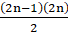= n(2n-1)

∴ it is multiple of n

Hence, sum of n consecutive odd numbers is divisible by n

Question 6.

Is 111 + 211 + 311 + 411 divisible by 5? Explain.

Given, 111 + 211 + 311 + 411

We need to find out the given number is divisible by 5

No, the given number cannot be divisible by 5

Explanation:

For a number to be divisible by 5, it should end with 0 or 5.

Hence, the given number cannot be divisible by 5

Question 7.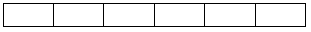Find the number of rectangles of the given figure?

Given, a rectangle grid

We need to find out the number of rectangles in the figure

⇒ 1 + 2 + 3 + 4 + 5 + 6 = 21

Hence, 21 rectangles are there in the given figure.

Question 8.

Rahul’s father wants to deposit some amount of money every year on the day of Rahul’s birthday. On his 1st birth day Rs.100, on his 2nd birth day Rs.300, on his 3rd birth day Rs.600, on his 4th birthday Rs.1000 and so on. What is the amount deposited by his father on Rahul’s 15th birthday.

Answer: Money deposited by Rahul's father is as follows:

100, 300, 600, 1000

They are in following series

100 + 1 × 0, 100 + 2 × 100, 300 + 3 × 100, 600 + 4 × 100

Multiplication numbers 1, 2, 3 & 4 represent the age Rahul is turning.

The multiplication factor is previous year deposit.

If we say, n is number of years, Rahul is turning and T1 = 100. Then the series can be re written as (T0 = 0)

T1 + n × T0 ,

T2 = T1 + n × 100,

T3 = T2 + n × 100 = T1 + (n-1) × 100 + n × 100

T4 = T3 + n × 100 = T1 + (n - 2) × 100 + (n - 1) × 100 + n × 100

....

T15 = T14 + 15 × 100

= 1 × 100 + 2 × 100 + 3 × 100 + ........+ 15 × 100

All the terms after T1 are in AP where, nth term is sum of n terms of the AP where

a = 100 and d = 100 n = 15

Hence T15 =

= n(2a + (n-1)d)/2

= 15(200 + 14 × 100)/2

= 15 × 800

= 12000

Question 9.

Find the sum of integers from 1 to 100 which are divisible by 2 or 5.

Given, Sum of integers from 1 to 100

Need to find out the numbers which are divisible by 2 or 5.

⇒The numbers which are divisible by 2 are 2,4,6….100

And the numbers which are divisible by 5 are 5,10,15…..100

⇒ Sum numbers are repeated twice hence, we need to take the LCM of 2 and 5 which is 10

∴ sum of numbers which are divisible by 10 are 10,20……100

⇒ Now, Sum of integers form 1 to N =∴ Sum of integers divisible by 2 or 5 from 1 to 100 = Sum of integers from 1 to 100 divisible by 2 + Sum of integers from 1 to 100 divisible by 5 – Sum of integers from 1 to 100 divisible by 10

= (2 + 4….. + 100) + (5 + 10 + ….. + 100)-(10 + 20 + …… + 100)

= 2(1 + 2 + … + 50) + 5(1 + 2 + …. + 20)-10(1 + 2 + ….. + 10)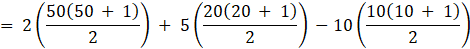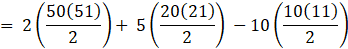= (50 × 51) + (5 × 10 × 21)-(5 × 10 × 11)

= 2550 + 1050-550

= 3050

Hence, the sum of integers from 1 to 100 which are divisible by 2 or 5 is 3050

Question 10.

Find the sum of integers from 11 to 1000 which are divisible by 3.

Given, Sum of integers from 11 to 1000

we need to find out the numbers which are divisible by 3

⇒ Now, Sum of integers form 1 to N =⇒ Sum of integers divisible by 3 from 11 to 1000 = Sum of integers divisible by 3 from 1 to 1000 – Sum of integers divisible by 3 from 1 to 10

= (3 + 6 + 9………. + 999) – (3 + 6 + …. + 9)

= 3(1 + 2 + 3…… + 333)-3(1 + 2 + .. + 3)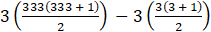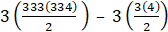= 3(333(167)) – 3(6)

= 3(55611)-18

= 166833-18

= 166815

Hence, the sum of integers from 11 to 1000 which are divisible by 3 is 166815.

## MATHS VIDEOS